ExetoolsC++ to masm conversion
 Register Forum Rules FAQ Calendar Mark Forums Read

 Notices https://forum.exetools.com This is the ONLY ONE domain that we use. Follow @exetools on Twitter and send me a message, I will choose whether to send the invitation code. Any password problems please mailto: 883600(at)qq(dot)com

#1
 ZeocrackFriend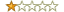Join Date: Sep 2022 Posts: 17 Rept. Given: 0 Rept. Rcvd 0 Times in 0 Posts Thanks Given: 63 Thanks Rcvd at 0 Times in 0 Posts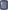C++ to masm conversion

Dear all
What could be the easiest source in masm for the below c++ code?

For i=222222 to 999999
C1=i
C2=i
C3=i
C4=i
C5=i
C6=i
Calcul=C1^6+C2^6+C3^6+C3¨6+C4^6+C5^6+C6^6
if Calcul=serial then
Msgbox("sérial" & i)

Exit
end if
Next i
#2
 chantsVIP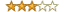Join Date: Jul 2016 Posts: 648 Rept. Given: 21 Rept. Rcvd 43 Times in 26 Posts Thanks Given: 593 Thanks Rcvd at 977 Times in 443 Posts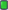Lol that is Visual Basic code not C++. So the carrot operator ^ represents exponentiation.

Exponentiation by a positive integer constant is best done by exponentuation by squaring most efficient by finding an addition chain which minimizes the number of multiplications and additions or subtractions. 6=4+2 or 6=8-2 will both work but 4+2 is more efficient

So you use IMUL to multiply by itself once, store the square the multiply the result by itself again, and multiply those together to get the 6th power. Accumulate with ADD and use a counter register initialized to 6 which decreases with DEC each time though the loop and check loop exit condition with jump if zero JZ. This is a very basic assembly task if you've learned it. Addition chains are a more advanced topic if your exponents are huge e.g. in cryptography. There is no known efficient way to find them interestingly enough
 The Following 2 Users Say Thank You to chants For This Useful Post: tonyweb (10-02-2022), Zeocrack (10-02-2022)
#3
 ZeocrackFriendJoin Date: Sep 2022 Posts: 17 Rept. Given: 0 Rept. Rcvd 0 Times in 0 Posts Thanks Given: 63 Thanks Rcvd at 0 Times in 0 PostsThank you. But the issue is we need a six digit number for which the equals to the sum of each 6th square. There seems more math.
#4
 ZeocrackFriendJoin Date: Sep 2022 Posts: 17 Rept. Given: 0 Rept. Rcvd 0 Times in 0 Posts Thanks Given: 63 Thanks Rcvd at 0 Times in 0 PostsThe below C++ code can solve the problem. But need to convert it in simple masm.

#include

int main(void)
{
int a,b,c,d,e,f;
int n;

for (a=2; a<=9; a++)
for (b=2; b<=9; b++)
for (c=2; c<=9; c++)
for (d=2; d<=9; d++)
for (e=2; e<=9; e++)
for (f=2; f<=9; f++)
{
n = 100000*a + 10000*b + 1000*c + 100*d + 10*e +f;
if (a*a*a*a*a*a + b*b*b*b*b*b + c*c*c*c*c*c + d*d*d*d*d*d + e*e*e*e*e*e + f*f*f*f*f*f == n)
{
printf("se! %i\n", n);
return 0;
}
}
}
#5
 chantsVIPJoin Date: Jul 2016 Posts: 648 Rept. Given: 21 Rept. Rcvd 43 Times in 26 Posts Thanks Given: 593 Thanks Rcvd at 977 Times in 443 PostsMight I suggest godbolt.org. Most here could handcraft this, but there us no need. How you can do it is using that website which shows the generated assembly code fir many C++ compilers, take your choice.
 The Following User Says Thank You to chants For This Useful Post: Zeocrack (10-02-2022)
#6
 te\$terFriendJoin Date: Feb 2013 Posts: 62 Rept. Given: 23 Rept. Rcvd 6 Times in 5 Posts Thanks Given: 19 Thanks Rcvd at 25 Times in 12 PostsYou may try gcc -S in order to get asm code
 The Following User Says Thank You to te\$ter For This Useful Post: Zeocrack (10-02-2022)
#7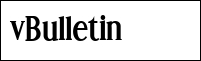Insid3CodeFamily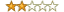Join Date: May 2013 Location: Algeria Posts: 85 Rept. Given: 47 Rept. Rcvd 60 Times in 30 Posts Thanks Given: 24 Thanks Rcvd at 107 Times in 57 PostsHi,
Using MSVC to compile "C snippet" and generate masm listing code:

Code:
```	.686p
.mmx
.xmm
.model flat, stdcall
option casemap :none

printf PROTO c :vararg
EXTRN getchar:PROC

includelib msvcrt.lib

.const
result DB 'se! %i', 0aH, 00H

.Code
tv273 = -52						; size = 4
_a\$ = -48						; size = 4
tv529 = -44						; size = 4
tv440 = -40						; size = 4
tv351 = -36						; size = 4
tv326 = -32						; size = 4
tv302 = -28						; size = 4
tv544 = -24						; size = 4
_b\$ = -20						; size = 4
_c\$ = -16						; size = 4
_d\$ = -12						; size = 4
_e\$ = -8						; size = 4
_f\$ = -4						; size = 4
start	PROC

; 4    : {

push	ebp
mov	ebp, esp
sub	esp, 52					; 00000034H
push	ebx
push	esi

; 5    : int a,b,c,d,e,f;
; 6    : int n;
; 7    :
; 8    : for (a=2; a<=9; a++)

mov	DWORD PTR _a\$[ebp], 2
mov	DWORD PTR tv544[ebp], 222222		; 0003640eH
push	edi
\$LL37@main:

; 9    : for (b=2; b<=9; b++)

mov	ecx, DWORD PTR _a\$[ebp]
mov	eax, ecx
imul	eax, ecx
imul	eax, ecx
imul	eax, ecx
imul	eax, ecx
imul	eax, ecx
mov	DWORD PTR tv273[ebp], eax
mov	eax, DWORD PTR tv544[ebp]
mov	DWORD PTR _b\$[ebp], 2
mov	DWORD PTR tv529[ebp], eax
\$LL38@main:

; 10   : for (c=2; c<=9; c++)

mov	eax, DWORD PTR _b\$[ebp]
mov	esi, eax
imul	esi, eax
imul	esi, eax
imul	esi, eax
imul	esi, eax
imul	esi, eax
mov	eax, DWORD PTR tv529[ebp]
mov	DWORD PTR _c\$[ebp], 2
mov	DWORD PTR tv440[ebp], eax
\$LL39@main:

; 11   : for (d=2; d<=9; d++)

mov	eax, DWORD PTR _c\$[ebp]
mov	edx, eax
imul	edx, eax
imul	edx, eax
imul	edx, eax
imul	edx, eax
imul	edx, eax
mov	eax, DWORD PTR tv440[ebp]
mov	DWORD PTR _d\$[ebp], 2
mov	DWORD PTR tv351[ebp], eax
\$LL40@main:

; 12   : for (e=2; e<=9; e++)

mov	eax, DWORD PTR _d\$[ebp]
mov	ecx, eax
imul	ecx, eax
imul	ecx, eax
imul	ecx, eax
imul	ecx, eax
imul	ecx, eax
mov	eax, DWORD PTR tv351[ebp]
mov	DWORD PTR _e\$[ebp], 2
mov	DWORD PTR tv326[ebp], eax
\$LL41@main:

; 13   : for (f=2; f<=9; f++)

mov	edi, DWORD PTR _e\$[ebp]
mov	eax, edi
imul	eax, edi
imul	eax, edi
imul	eax, edi
imul	eax, edi
imul	eax, edi
mov	edi, DWORD PTR tv326[ebp]
mov	DWORD PTR _f\$[ebp], 2
mov	DWORD PTR tv302[ebp], edi
\$LL4@main:

; 14   : {
; 15   : n = 100000*a + 10000*b + 1000*c + 100*d + 10*e +f;
; 16   : if (a*a*a*a*a*a + b*b*b*b*b*b + c*c*c*c*c*c + d*d*d*d*d*d + e*e*e*e*e*e + f*f*f*f*f*f == n)

mov	edi, DWORD PTR _f\$[ebp]
mov	ebx, edi
imul	ebx, edi
imul	ebx, edi
imul	ebx, edi
imul	ebx, edi
imul	ebx, edi
cmp	ebx, DWORD PTR tv302[ebp]
je	SHORT \$LN30@main

; 13   : for (f=2; f<=9; f++)

inc	DWORD PTR _f\$[ebp]
inc	DWORD PTR tv302[ebp]
push	9
pop	edi
cmp	DWORD PTR _f\$[ebp], edi
jle	SHORT \$LL4@main

; 12   : for (e=2; e<=9; e++)

inc	DWORD PTR _e\$[ebp]
add	DWORD PTR tv326[ebp], 10		; 0000000aH
cmp	DWORD PTR _e\$[ebp], edi
jle	SHORT \$LL41@main

; 11   : for (d=2; d<=9; d++)

inc	DWORD PTR _d\$[ebp]
add	DWORD PTR tv351[ebp], 100		; 00000064H
cmp	DWORD PTR _d\$[ebp], edi
jle	\$LL40@main

; 10   : for (c=2; c<=9; c++)

inc	DWORD PTR _c\$[ebp]
add	DWORD PTR tv440[ebp], 1000		; 000003e8H
cmp	DWORD PTR _c\$[ebp], edi
jle	\$LL39@main

; 9    : for (b=2; b<=9; b++)

inc	DWORD PTR _b\$[ebp]
add	DWORD PTR tv529[ebp], 10000		; 00002710H
cmp	DWORD PTR _b\$[ebp], edi
jle	\$LL38@main

; 5    : int a,b,c,d,e,f;
; 6    : int n;
; 7    :
; 8    : for (a=2; a<=9; a++)

add	DWORD PTR tv544[ebp], 100000		; 000186a0H
inc	DWORD PTR _a\$[ebp]
cmp	DWORD PTR tv544[ebp], 922222		; 000e126eH
jle	\$LL37@main

; 14   : {
; 15   : n = 100000*a + 10000*b + 1000*c + 100*d + 10*e +f;
; 16   : if (a*a*a*a*a*a + b*b*b*b*b*b + c*c*c*c*c*c + d*d*d*d*d*d + e*e*e*e*e*e + f*f*f*f*f*f == n)

jmp	SHORT \$LN17@main
\$LN30@main:

; 17   : {
; 18   : printf("se! %i\n", n);

push	DWORD PTR tv302[ebp]
push	OFFSET result
call	printf
pop	ecx
pop	ecx

; 19   : getchar();

call	getchar
\$LN17@main:

; 20   : return 0;
; 21   : }
; 22   : }
; 23   : }

pop	edi
pop	esi
xor	eax, eax
pop	ebx
leave
ret	0
start	ENDP
END```
You can compile it with MASM32...
Attached source and (binary size 1KB) clean asm code easy to debug...

https://www.sendspace.com/file/olce5h
Attached Files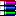release.rar (1.9 KB, 3 views)
__________________
Computer Forensics
#8
 ZeocrackFriendJoin Date: Sep 2022 Posts: 17 Rept. Given: 0 Rept. Rcvd 0 Times in 0 Posts Thanks Given: 63 Thanks Rcvd at 0 Times in 0 PostsQuote:
 Originally Posted by Insid3CodeHi, Using MSVC to compile "C snippet" and generate masm listing code: Code: ``` .686p .mmx .xmm .model flat, stdcall option casemap :none printf PROTO c :vararg EXTRN getchar:PROC includelib msvcrt.lib .const result DB 'se! %i', 0aH, 00H .Code tv273 = -52 ; size = 4 _a\$ = -48 ; size = 4 tv529 = -44 ; size = 4 tv440 = -40 ; size = 4 tv351 = -36 ; size = 4 tv326 = -32 ; size = 4 tv302 = -28 ; size = 4 tv544 = -24 ; size = 4 _b\$ = -20 ; size = 4 _c\$ = -16 ; size = 4 _d\$ = -12 ; size = 4 _e\$ = -8 ; size = 4 _f\$ = -4 ; size = 4 start PROC ; 4 : { push ebp mov ebp, esp sub esp, 52 ; 00000034H push ebx push esi ; 5 : int a,b,c,d,e,f; ; 6 : int n; ; 7 : ; 8 : for (a=2; a<=9; a++) mov DWORD PTR _a\$[ebp], 2 mov DWORD PTR tv544[ebp], 222222 ; 0003640eH push edi \$LL37@main: ; 9 : for (b=2; b<=9; b++) mov ecx, DWORD PTR _a\$[ebp] mov eax, ecx imul eax, ecx imul eax, ecx imul eax, ecx imul eax, ecx imul eax, ecx mov DWORD PTR tv273[ebp], eax mov eax, DWORD PTR tv544[ebp] mov DWORD PTR _b\$[ebp], 2 mov DWORD PTR tv529[ebp], eax \$LL38@main: ; 10 : for (c=2; c<=9; c++) mov eax, DWORD PTR _b\$[ebp] mov esi, eax imul esi, eax imul esi, eax imul esi, eax imul esi, eax imul esi, eax mov eax, DWORD PTR tv529[ebp] mov DWORD PTR _c\$[ebp], 2 mov DWORD PTR tv440[ebp], eax \$LL39@main: ; 11 : for (d=2; d<=9; d++) mov eax, DWORD PTR _c\$[ebp] mov edx, eax imul edx, eax imul edx, eax imul edx, eax imul edx, eax imul edx, eax mov eax, DWORD PTR tv440[ebp] mov DWORD PTR _d\$[ebp], 2 mov DWORD PTR tv351[ebp], eax \$LL40@main: ; 12 : for (e=2; e<=9; e++) mov eax, DWORD PTR _d\$[ebp] mov ecx, eax imul ecx, eax imul ecx, eax imul ecx, eax imul ecx, eax imul ecx, eax mov eax, DWORD PTR tv351[ebp] mov DWORD PTR _e\$[ebp], 2 mov DWORD PTR tv326[ebp], eax \$LL41@main: ; 13 : for (f=2; f<=9; f++) mov edi, DWORD PTR _e\$[ebp] mov eax, edi imul eax, edi imul eax, edi imul eax, edi imul eax, edi imul eax, edi mov edi, DWORD PTR tv326[ebp] mov DWORD PTR _f\$[ebp], 2 mov DWORD PTR tv302[ebp], edi \$LL4@main: ; 14 : { ; 15 : n = 100000*a + 10000*b + 1000*c + 100*d + 10*e +f; ; 16 : if (a*a*a*a*a*a + b*b*b*b*b*b + c*c*c*c*c*c + d*d*d*d*d*d + e*e*e*e*e*e + f*f*f*f*f*f == n) mov edi, DWORD PTR _f\$[ebp] mov ebx, edi imul ebx, edi imul ebx, edi imul ebx, edi imul ebx, edi imul ebx, edi add ebx, DWORD PTR tv273[ebp] add ebx, esi add ebx, edx add ebx, ecx add ebx, eax cmp ebx, DWORD PTR tv302[ebp] je SHORT \$LN30@main ; 13 : for (f=2; f<=9; f++) inc DWORD PTR _f\$[ebp] inc DWORD PTR tv302[ebp] push 9 pop edi cmp DWORD PTR _f\$[ebp], edi jle SHORT \$LL4@main ; 12 : for (e=2; e<=9; e++) inc DWORD PTR _e\$[ebp] add DWORD PTR tv326[ebp], 10 ; 0000000aH cmp DWORD PTR _e\$[ebp], edi jle SHORT \$LL41@main ; 11 : for (d=2; d<=9; d++) inc DWORD PTR _d\$[ebp] add DWORD PTR tv351[ebp], 100 ; 00000064H cmp DWORD PTR _d\$[ebp], edi jle \$LL40@main ; 10 : for (c=2; c<=9; c++) inc DWORD PTR _c\$[ebp] add DWORD PTR tv440[ebp], 1000 ; 000003e8H cmp DWORD PTR _c\$[ebp], edi jle \$LL39@main ; 9 : for (b=2; b<=9; b++) inc DWORD PTR _b\$[ebp] add DWORD PTR tv529[ebp], 10000 ; 00002710H cmp DWORD PTR _b\$[ebp], edi jle \$LL38@main ; 5 : int a,b,c,d,e,f; ; 6 : int n; ; 7 : ; 8 : for (a=2; a<=9; a++) add DWORD PTR tv544[ebp], 100000 ; 000186a0H inc DWORD PTR _a\$[ebp] cmp DWORD PTR tv544[ebp], 922222 ; 000e126eH jle \$LL37@main ; 14 : { ; 15 : n = 100000*a + 10000*b + 1000*c + 100*d + 10*e +f; ; 16 : if (a*a*a*a*a*a + b*b*b*b*b*b + c*c*c*c*c*c + d*d*d*d*d*d + e*e*e*e*e*e + f*f*f*f*f*f == n) jmp SHORT \$LN17@main \$LN30@main: ; 17 : { ; 18 : printf("se! %i\n", n); push DWORD PTR tv302[ebp] push OFFSET result call printf pop ecx pop ecx ; 19 : getchar(); call getchar \$LN17@main: ; 20 : return 0; ; 21 : } ; 22 : } ; 23 : } pop edi pop esi xor eax, eax pop ebx leave ret 0 start ENDP END``` You can compile it with MASM32... Attached source and (binary size 1KB) clean asm code easy to debug... External link: https://www.sendspace.com/file/olce5h

Hi insid3code

Thanks. Can you explain a little more so that I can try my own,

Thanks
#9Insid3CodeFamilyJoin Date: May 2013 Location: Algeria Posts: 85 Rept. Given: 47 Rept. Rcvd 60 Times in 30 Posts Thanks Given: 24 Thanks Rcvd at 107 Times in 57 PostsMSVC compiler can generate from your "c" snippet masm listing..
to generate this masm file:
cl.exe /I"include" /D "WIN32" /X /c /O1 /Zl /Os /GF /EHsc /GS- /W4 /Gd /FAs /Fa"asmX86" "main.c"

You can use the generated masm listing directly under MASM32.
__________________
Computer Forensics
 The Following User Says Thank You to Insid3Code For This Useful Post: Zeocrack (10-04-2022)

 Tags source coThread ToolsShow Printable VersionEmail this Page Display ModesLinear ModeSwitch to Hybrid ModeSwitch to Threaded ModePosting Rules You may not post new threads You may not post replies You may not post attachments You may not edit your posts BB code is On Smilies are On [IMG] code is On HTML code is On Forum Rules

All times are GMT +8. The time now is 01:30.

 Aaron's homepage - TopAlways Your Best Friend: Aaron, JMI, ahmadmansoor, ZeNiX, chessgod101
( 1998 - 2023 )